Next: Effective Interactions (I) Up: MANY-NUCLEON SYSTEMS Previous: MANY-NUCLEON SYSTEMS

General Discussion of the Nuclear Many-Body Problem

We begin our discussion of many-nucleon systems by (again) identifying the most important degrees of freedom and writing down the relevant Hamiltonian. Contrary to methods used at a finer level (quarks and gluons) we use here the Hamiltonian picture instead of the Lagrangian density; this is so because most of the analysis can be done in the framework of the standard quantum mechanics, without necessity of applying methods of the quantum field theory. Nevertheless, we shall express our many-body Hamiltonian in the language of the fermion creation and annihilation operators, which is very convenient in any theory that involves many identical particles obeying specific exchange symmetries.

In order to simplify the discussion we disregard the three-body NNN piece of the interaction between the nucleons, and thus the most general Hamiltonian of a many-nucleon system can be written as,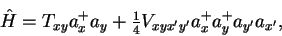(57)

where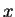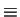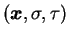,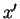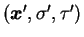, etc., are the space-spin-isospin variables, and the summation-integration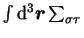is implied for every pair of repeated indices. Following the standard notation, we put the space-spin-isospin arguments as indices of the kinetic energy,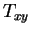, potential energy,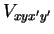, and the creation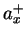and annihilation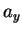operators. We assume that the two-body potential energy operator is antisymmetrized,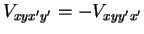.

We can now estimate the order of complication involved in a many-nucleon system. Let us assume that fields(i.e., the s.p. wave functions) have to be known at about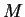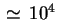space-spin-isospin points. The estimate involves, say, about 20 points of a 1fm lattice in each of the three spatial direction, and four spin-isospin components. The 1fm lattice may seem to be grossly insufficient to describe a system where a typical s.p. kinetic energy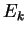is of the order of 50MeV, and thus involves typical s.p. momenta of nucleons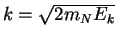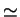300MeV1.3fm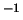(0.7fm). However, typical scale at which total densities of nucleons vary in a nucleus, are of the order of 2-3fm, so the 1fm lattice is a barely sufficient, but fair compromise to describe a system having the total size (including the asymptotic peripheral region) of at least 20fm.

The fermion Fock space, i.e., the complete Hilbert space that is relevant to describe a system of many identical fermions, has the dimensionality of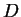=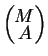, which is equal to the number of ways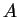fermions can be distributed onsites. For the=10 systems, which at present can still be treated within the GFMC method, Sect. 3.4, we thus obtain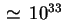. On the one hand, this number illustrates the power of the existing theoretical descriptions; on the other hand, it explains why it is so difficult to go any further. For example, for a heavy=200 nucleus, the dimensionality reaches 10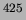. Therefore, it is neither conceivable nor sensible to envisage any exact methods for heavy nuclei.

One has to bear, however, in mind that the physics of a heavy nucleus does not really require such a detailed knowledge of any of its states. To see this, let us consider the energy of an arbitrary state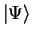as given by the average value of the Hamiltonian,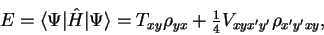(58)

where the one- and two-body density matrices are defined as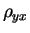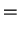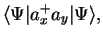(59)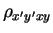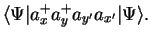(60)

Both density matrices are Hermitian,=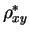and=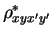, and the (fermion) two-body density matrix is antisymmetric with respect to exchanging its first two, or last two arguments,=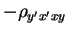=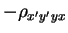. Hence the total energy of an arbitrary many-fermion state, described by two-body interactions, is determined by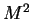+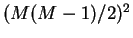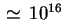real parameters for(independently of). Even when the three-body interactions are taken into account, this number grows only'' to 10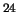. This shows explicitly, that the information contained in a many-fermion nuclear state is, in fact, much smaller than the total dimensionality of the Hilbert space, or in other words, only very specific states from this Hilbert space are relevant.

Unfortunately, the presented counting rules, based on the analysis of density matrices, do not help in obtaining practical solutions for many-body problems. The reason for that is the never-solved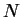-representability problem Col63,Col00, namely, the question: which of the four-index matrices are two-body density matrices of many-fermion states, and which are not. Indiscriminate variation of Eq. (58) over the density matrices (to look for the ground state) is, therefore, inappropriate. Hence, we are back to square one, i.e., we have to anyhow consider the full Hilbert space to look for correct many-fermion states, even if we know that this constitutes an enormous waste of effort. New bright ideas to solve the-representability problem in nuclear-physics context are very much needed. Before this is achieved, we are bound to look for methods judiciously reducing the dimensionality of the many-body problems. There are two main avenues to do so, which we briefly describe in the next two Sections.Next: Effective Interactions (I) Up: MANY-NUCLEON SYSTEMS Previous: MANY-NUCLEON SYSTEMS
Jacek Dobaczewski 2003-01-27